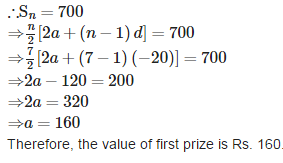# Test: Arithmetic Progressions - 4

## 25 Questions MCQ Test Mathematics (Maths) Class 10 | Test: Arithmetic Progressions - 4

Description
This mock test of Test: Arithmetic Progressions - 4 for Class 10 helps you for every Class 10 entrance exam. This contains 25 Multiple Choice Questions for Class 10 Test: Arithmetic Progressions - 4 (mcq) to study with solutions a complete question bank. The solved questions answers in this Test: Arithmetic Progressions - 4 quiz give you a good mix of easy questions and tough questions. Class 10 students definitely take this Test: Arithmetic Progressions - 4 exercise for a better result in the exam. You can find other Test: Arithmetic Progressions - 4 extra questions, long questions & short questions for Class 10 on EduRev as well by searching above.
QUESTION: 1

### Progressions with equal common difference are known as

Solution:

Progressions with equal common difference are known as Arithmetic Progression.

QUESTION: 2

### The first term of an A.P., if its Sn = n2+2n is

Solution: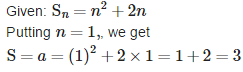QUESTION: 3

### If the second term of an AP is 13 and its fifth term is 25, then its 7th term is

Solution: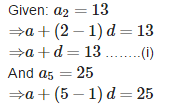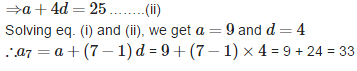QUESTION: 4

In an A.P., if am = 1/n and an = 1/m, then amn =

Solution: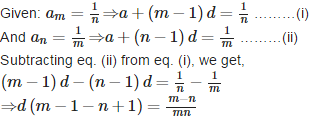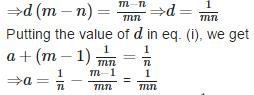∴ amn = a + (mn - 1) d = 1/mn + (mn - 1)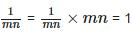QUESTION: 5

The first term of an AP is 5, the last term is 45 and the sum is 400. The number of terms is

Solution: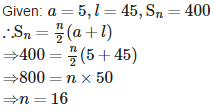QUESTION: 6

The value of ‘k’ for which the numbers x, 2x + k, 3x + 6 are in A.P. is

Solution: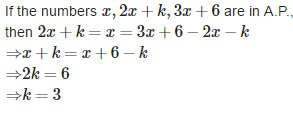QUESTION: 7

If the common difference of an A.P. is 5, then the value of a20 − a13 is

Solution:

Given: a20 - a13 and d = 5
⇒ a20 - a13 = a + (20 - 1) d - [a + (13 - 1) d] = a + (20 - 1) x 5 - [a + (13 - 1) x 5]
⇒ a20 - a13 - a = a + 95 - [a + 60]
= a+95 - a - 60 = 35

QUESTION: 8

Two APs have the same common difference. The difference between their 100th terms is 100, then the difference between their 1000th terms is

Solution:
QUESTION: 9

The common difference of the A.P whose Sn = 3n2+ 2n is

Solution: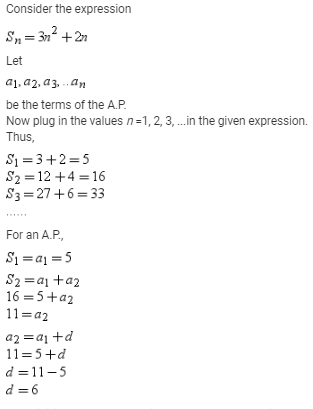Thus, initial term of the A.P. is 5 and the common difference is 6.

QUESTION: 10

The sum of (a + b), (a – b), (a – 3b), …….. to 22nd term is

Solution:

First Term =  a+b
Second Term =  a-b
Common Difference is a-b-a-b = -2b.

Summation of 22 terms of an A.P. is
n/2 [ 2a + (n-1)d ]
22/2 [2 ( a+b) + (22-1)-2b ]
11  [ 2a+2a-b + (21)-2b  ]
11  [  2a+2b - 42b ]
11  [ 2a - 40b ]
22a - 40b.

So, the summation of given A.P. for 22 terms is 22a - 40b

QUESTION: 11

The next term of the A.P. √18 , √32 and √50 is

Solution: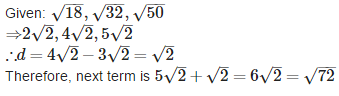QUESTION: 12

If 9 times the 9th term of an A.P. is equal to 11 times the 11th term , then its 20th term is

Solution: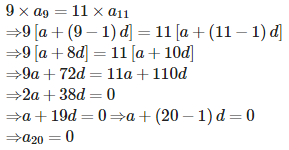QUESTION: 13

The 17th term of an AP exceeds its 10th term by 7, then the common difference is

Solution: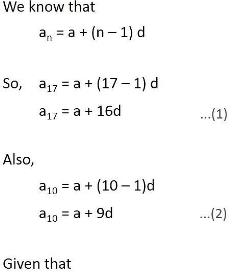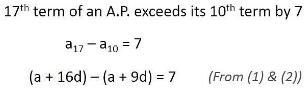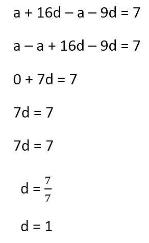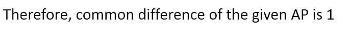QUESTION: 14

The sum of three terms of an A.P. is 72, then its middle term is

Solution:

Let the middle term be a, then first term is a−d and next term is a+d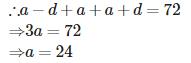QUESTION: 15

The sum of odd numbers between 0 and 50 is

Solution:

Odd numbers between 0 and 50 are 1, 3, 5, 7, ………, 49 Here a = 1,d = 3−1 = 2 and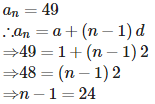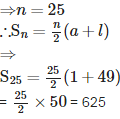QUESTION: 16

If a, b and c are in A.P., then the relation between them is given by

Solution:

If a, b and c are in A.P., then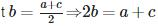QUESTION: 17

The 7th term from the end of the A.P. – 11, – 8, – 5, ……., 49 is

Solution: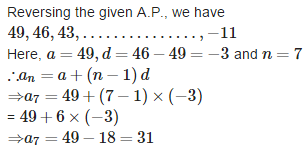QUESTION: 18

The number of three digit numbers divisible by 7 is

Solution:

Three digits numbers divisible by 7 are 105, 112, 119,.........., 994
Here, a= 105, d = 112 - 105 = 7, an = 994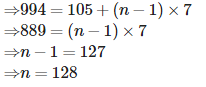QUESTION: 19

The first and last terms of an A.P. are 1 and 11. If their sum is 36, then the number of terms will be

Solution: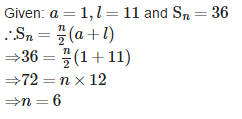QUESTION: 20

If 1 + 4 + 7 + ……. + k = 287, then the value of ‘k’ is

Solution: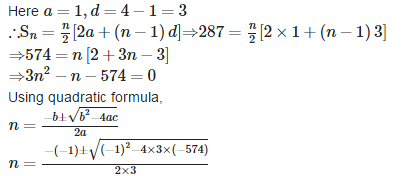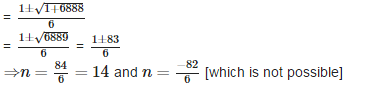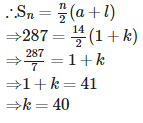QUESTION: 21

If the angles of a right angled triangle are in A.P. then the angles of that triangle will be

Solution:

Let the three angles of a triangle be a - d, a and a + d.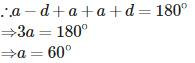Therefore, one angle is of 60° and other is 90° (given). Let third angle be x° . then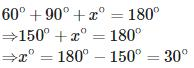Therefore the angles of the right angled triangle are 30°, 60°, 90°.

QUESTION: 22

The 10th term of an A.P. 2, 7, 12, …….. is

Solution: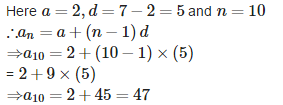QUESTION: 23

If a= 4 and an = 4an−1+3, n  >1, then the value of a4 is

Solution: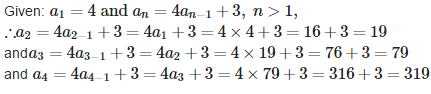QUESTION: 24

The number of terms of the A.P. 5, 8, 11, 14, ……. to be taken so that the sum is 258 is

Solution: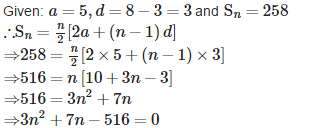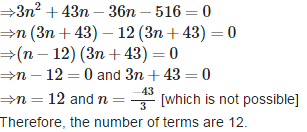QUESTION: 25

A sum of Rs.700 is to be used to award 7 prizes. If each prize is Rs.20 less than its preceding prize, then the value of the first prize is

Solution:

Let the first prize be a.
The seven prizes form an AP with first term a and common difference d = −20
Now the sum of all seven prizes = Rs. 700